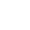## 海豚网游加速器# 台服R2 Online精灵&召唤法书出处整理攻略

A(弱)>>>>>>>>R(强) 精英&BOSS看地区怪强弱

@以下召喚

阿克拉岛(精灵&召唤出生地)

硬刺龟 D 召唤嗜血曼陀罗

巴利安猩猩 E 召唤生命图腾

阿尔岩猩猩 E 召唤防御图腾

阿尼巴尔勇士F 召唤哥布林炸弹

娜迦刀兵 F 召唤魔力曼陀罗

娜迦女王 精英 召唤嗜血曼陀罗

齐天大圣 BOSS 海神之怒

猛虎指挥官 BOSS 地狱烈焰

@地下城

不死地牢

巫妖王(3F) BOSS 陨石术

烈焰之塔

烈火匹斯(4F)BOSS 地狱烈焰

@以下精灵

阿克拉岛(精灵&招换 出生地)

阿尔岩猩猩 E 驱散魔法(I)

卡尼巴尔勇士F 厄运之蚀(I)

猛虎士兵 K 烈焰风暴(I)

复仇之地

地精 C 神圣铠甲(I)

地精弓箭手 C 魔法盾(I)

棕熊 B 石化皮肤(I)

蜘蛛 D 蛛网术(I)

克里亚特猎鸟蛛 精英 蛛网术(I)

双足翼龙 精英 负重增加(I)

黑暗大陆

蜥蜴 B 魔法盾(I)

小恶魔 C 剑术精通(I)

死亡克鲁巫师C 剑术精通(I) 火球术(I) 冰箭术(I)

巨鹰 E 神圣铠甲(I)

鳞刺鱼人 F 剑术精通(II)

拜伦领地

丛林拉米亚 C 法力恢复(I)

小仙子 F 雷电召唤(I) 传送术

罗敦领地

牛头人勇士 精英 沉默

@地下城

不死地牢

骷髅骑士(1F)E 剑术精通(I)

巫师(3F) H 祝福光环(I)

帝王墓穴

梦魔(1F) H 剑术精通(III)

吸血鬼女王(2F) G 厄运之触(I) 雷电召唤(I)

黑暗洞窟

史莱姆(1F) B 石化皮肤(I)

哥不林矿工(1F) D 剑术精通(I)

洞穴蜘蛛(2F)E 蛛网术(I)

骷髅骑士(3F)E 剑术精通(I)

进化中的狼蛛(3F) F 蛛网术(I)

黑暗追踪者(4F) I 侵蚀火焰(I)

女巫(4F) I 驱散魔法(I)

烈焰塔

火骷髅(1F) E 驱散魔法(I)

消亡的骷髅(1F) E 雷电召唤(I)

燃烧的食尸鬼(1F) F 厄运之蚀(I)

泰坦兽人战士(2F) F 剑术精通(II)

火狼(2F) F 增加负重(I)

炼狱女巫(4F)K 烈焰风暴(I)

埃吉耳洞窟

冰霜沙罗曼蛇(1F) G 暴风雪(I) 冰箭术(I)

原始海盗洞窟

海盗诅咒师(2F) Q 侵蚀火焰(III) 剑术精通(IV) 烈焰风暴(II)

埃塔斯洞窟

恶魔史雷德(内) L 厄运之蚀(I) 剧毒之云

阿特拉茜亚(内) BOSS 剑术精通(III)

米泰欧斯

双足翼龙拉伊德(外) BOSS 烈焰风暴(I)

米泰欧斯诅咒师(内) O 剑术精通(III) 祝福光环(I) 烈焰风暴(I)

米泰欧斯之塔

血之咒术师 P 石化皮肤(II)

火焰术士 P 祝福光环(II)

猛毒莫斯 P 增加负重(II)

深海青龙 P 法力恢复(II) 魔法盾(II)

大地君主 BOSS 蛛网术(II)

猛毒君主 BOSS 厄运之蚀(III)

烈焰暴君 BOSS 烈焰风暴(II)

荣光圣殿

变异的奎特普特 O 剑术精通(IV)

堕落的双足翼龙 P 厄运之蚀(II)

嗜血天蝎 P 剑术精通(IV)

红色尖刺潘瑟 P 法力恢复(II)

变质的格雷芬特 P 蛛网术(II)

黄泉咒术师 Q 烈焰风暴(II)

盈月遗迹

大地双足翼龙 R 魔法盾(II)

暴走的双头巨人 R 增加负重(II)

城堡魔像 R 石化皮肤(II) 祝福光环(II)

堕落的暗黑精灵 R 侵蚀火焰(III)

战场食人魔 R 烈焰风暴(III)

漆黑夜之梦魔 R 剑术精通(IV) 蛛网术(II) 厄运之蚀(III)

塔纳托斯 BOSS 侵蚀火焰(II)

骑士团长拉尔卡 BOSS 烈焰风暴(II)

海豚网游加速器是一款专门帮助游戏玩家解决游戏卡顿、延时高、掉线、登陆不上等问题的游戏辅助软件。通过遍布海内外的加速节点，以动态路由调整、7x24小时全时段网络检测、数据以最优路径中转等技术，确保每一位玩家的游戏通顺!

点击下载游戏加速器>>>